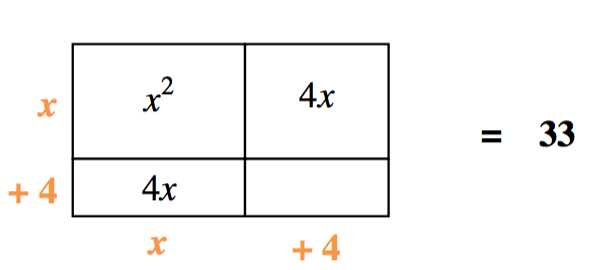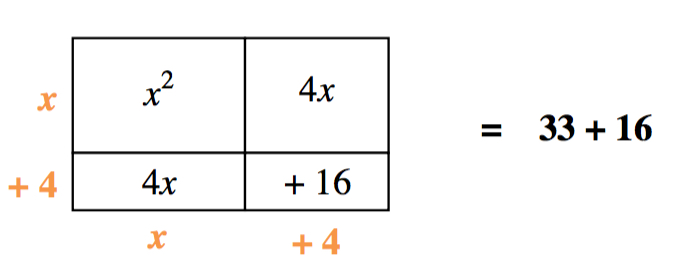### Home > INT2 > Chapter 7 > Lesson 7.2.3 > Problem7-118

7-118.

Solve each of the following by completing the square.

1. $0 = x^2 + 8x - 33$

1. $x^2 - 2x + 5 = 0$

1. $x^2 - 7x - 3 = 0$

Use an area model if you need help.

Equation: Generic rectangle = 33. Rectangle: Left edge labeled, x, + 4, bottom edge labeled, x, + 4. Interior labeled: top left, x squared, top right, 4, x, bottom left, 4, x, bottom right, blank

Added to rectangles, Interior bottom right, + 16. Added to equation, right side, + 16.

$(x+4)^{2}=49$

$x+4=\pm7$

$x=-11\text{ or }3$